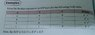# Boolean's Algebra - SOP

• desmond iking

#### desmond iking

Can someone please explain why the F( X, Y ) is XY' + XY ? IMO , it is X'Y' + X'Y +XY' +XY ... or the author simplified it to become XY' + XY ? how to simplify it?

#### Attachments

•IMG_20141215_212410.jpg
28 KB · Views: 406
Use boolean algebra to reduce it. In boolean algebra X+X' = 1 and use algebra to extract common factors.

Is this a homework assignment?

jedishrfu said:
Use boolean algebra to reduce it. In boolean algebra X+X' = 1 and use algebra to extract common factors.

Is this a homework assignment?
no, i am studying myself during the holiday.

•jedishrfu

Boolean algebra rules are the same as ordinary algebra with the added concepts X=X'=1 and X.X'=0 and X=0 means X=false and X=1 means X=true. Engineers start with a truth table for a circuit, construct boolean expressions, reduce them down using boolean algebra ruls and then construct a circuit from the reduced expressions.

jedishrfu said:

Boolean algebra rules are the same as ordinary algebra with the added concepts X=X'=1 and X.X'=0 and X=0 means X=false and X=1 means X=true. Engineers start with a truth table for a circuit, construct boolean expressions, reduce them down using boolean algebra ruls and then construct a circuit from the reduced expressions.
i only managed to reduce it to XY +Y' +X'Y
here's my working:
(X'Y') + (X'Y) + (XY') + (XY)
=XY +Y'(X' +X ) +X'Y
= XY +Y' +X'Y

what about the 1st and 3rd term? they have a common Y.

jedishrfu said:
what about the 1st and 3rd term? they have a common Y.

continue form the previous post , i still didnt get the ans
XY +Y' +X'Y = Y(X +X') +Y' = Y+Y'

desmond iking said:
Can someone please explain why the F( X, Y ) is XY' + XY ?
Hi Desmond. No one can explain it. It's a misprint.It is just the exclusive OR function, X'Y + XY'. It doesn't simplify, except you can write it as X ⨁ Y.
Good luck with your self-study. http://thumbnails112.imagebam.com/37333/0363e9373324851.jpg [Broken]

Last edited by a moderator:
•desmond iking
I noticed that theF(X , Y) is the outcome of the addition of the last two. *correct me if I m wrong* , why it only involve the addition of last two term? Why not also the first two terms??

desmond iking said:
I noticed that theF(X , Y) is the outcome of the addition of the last two. *correct me if I m wrong* , why it only involve the addition of last two term? Why not also the first two terms??
It's a misprint. F(X,Y) is true when either of these is true: X'Y or XY'

•desmond iking